# Powers and roots

Operations with powers. Multiplication and division of powers.
Power of product of some factors. Power of a quotient (fraction). Raising of power to a power. Operations with roots. Arithmetical
root. Root of product of some factors. Root of quotient (fraction).
Raising of root to a power. Proportional change of degrees of a
root and its radicand. Negative, zero and fractional exponents
of a power. About meaningless expressions.

Operations with powers.

1. At multiplying of powers with the same base their exponents are added:

a m · a n =  a m + n .

2. At dividing of powers with the same base their exponents are subtracted: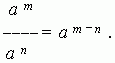3. A power of product of two or some factors is equal to a product of powers of these factors:

( abc ) n = a n · b n · c n

4. A power of a quotient (fraction) is equal to a quotient of powers of a dividend     (numerator) and a divisor (denominator):

( a / b ) n =  a n /  b n .

5. At raising of a power to a power their exponents are multiplied:

( a m ) n =  a m n .

All above mentioned formulas are read and executed in both  directions – from the left to the right and back.

E x a m p l e .  ( 2 · 3 · 5 / 15 ) 2 = 2 2 · 3 2 · 5 2 / 15 2 = 900 / 225 = 4 .

Operations with roots. In all below mentioned formulas a symbol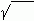means an arithmetical root ( all radicands are considered here only positive ).

1. A root of product of some factors is equal to a product of roots of these factors: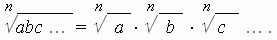2. A root of a quotient is equal to a quotient of roots  of a dividend and a divisor: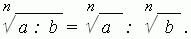3. At raising a root to a power it is sufficient to raise a radicand to this power: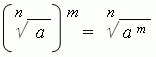4. If to increase a degree of a root by m times and to raise simultaneously its    radicand to the m -th power, the root value doesn’t change: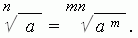5. If  to decrease a degree of a root by m times and to extract simultaneously the m -th degree root of the radicand,  the root value doesn’t change: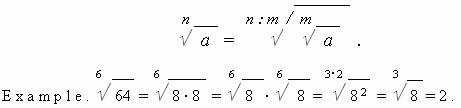Widening of the power notion. Till now we considered only natural exponents   of powers; but operations with powers and roots can result also to negative , zero and fractional exponents . All these exponents of powers require to be defined.

Negative exponent of a power. A power of some number with a negative (integer) exponent is defined as unit divided by the power of  the same number with the exponent equal to an absolute value of the negative exponent: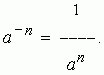Now the formula a m : a n = a m - n may be used not only if m is more than n , but also for a case if m is less than n .

E x a m p l e . a 4 : a 7 = a 4 - 7 = a - 3 .

If we want the formula a m : a n = a m - n to be valid at m = n we need the definition of zero exponent of a power.

Zero exponent of a power. A power of any non-zero number with zero exponent is equal to 1.

E x a m p l e s . 2 0 = 1,   ( 5 ) 0 = 1,   ( 3 / 5 ) 0 = 1.

Fractional exponent of a power. To raise a real number a to a power with an exponent m / n it is necessary to extract the n -th degree root  from the m -th power of this number a :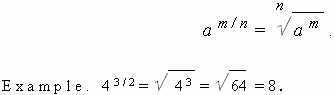About meaningless expressions. There are some expressions:

Case 1.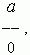where a 0 , doesn’t exist .

Really, if to assume that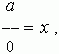where x – some number, then according

to the definition of a division we have: a = 0 · x ,  i.e. a = 0 ,  but this result

contradicts to the condition: a 0 .

Case 2.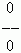is any number.

Really, if to assume that this expression is equal to some number x , then

according to the definition of a division:  0 = 0 · x . But this equality is valid

at any number x , which was to be proved.

Case 3.

If  to assume, that rules of operations with powers are spread to powers with

a zero base, then

0 0 is any number .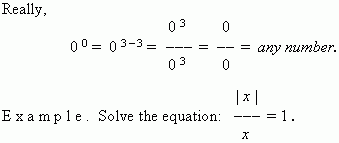S o l u t i o n . Consider the three main cases:

1) x = 0 this value doesn’t satisfy the equation  ( Why ? ) ;

2)  at x > 0 we receive: x / x = 1, i.e.1 = 1, hence, x – any number,

but  taking into consideration that in this case x > 0, the answer

is: x > 0 ;

3)  at x < 0 we receive: – x / x = 1, i.e.  –1 = 1,  and the answer is:

there is no solution in this case.

So, the answer: x > 0 .